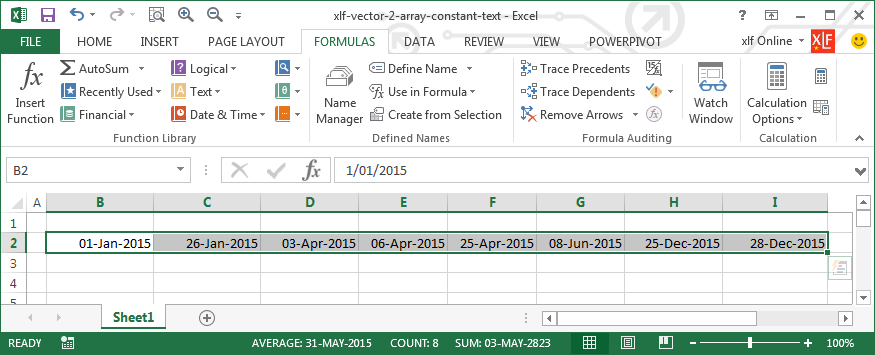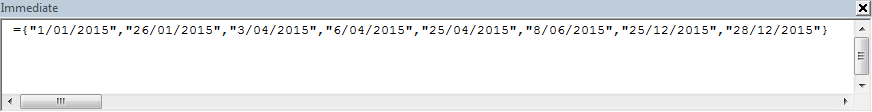# xlf | Vector2ArrayConstantText macro

## Vector2ArrayConstantText - about the macro

Description: returns a delimited array from an Excel selection.

The array format is suitable for use in an array constant. Example selection data, using dates, is shown in figure 1 The output to the Immediate Window appears in figure 2. The user can copy from the Immediate Window, and paste to the Refers to: panel of the Name dialog box.Fig 1: Worksheet selection - a row vector of datesFig 2: Immediate Window - Text string in array constant format.

## Vector2ArrayConstantText - the VBA code

Code 1: Macro - Vector2ArrayConstantText returns a delimited array from the Excel selection
Sub Vector2ArrayConstantText()
Dim NoRows As Long, NoCols As Long
Dim RowIndex As Long, ColIndex As Long
Const vbDQuote As String = """"
Dim ArrayV As String

NoRows = Selection.Rows.Count
NoCols = Selection.Columns.Count

'' Scalar element
If NoRows = 1 And NoCols = 1 Then Exit Sub

'' Column vector
If NoRows > 1 And NoCols = 1 Then
ArrayV = "={"
For RowIndex = 1 To NoRows
For ColIndex = 1 To NoCols
ArrayV = ArrayV & vbDQuote & Selection.Range("A1").Offset(RowIndex - 1, ColIndex - 1).Value & vbDQuote & ";"
If RowIndex + 1 = NoRows Then
ArrayV = ArrayV & vbDQuote & Selection.Range("A1").Offset(RowIndex, ColIndex - 1).Value & vbDQuote
NoCols = 0
Exit For
End If
Next ColIndex
Next RowIndex
End If

'' Row vector
If NoRows = 1 And NoCols > 1 Then
ArrayV = "={"
For RowIndex = 1 To NoRows
For ColIndex = 1 To NoCols
ArrayV = ArrayV & vbDQuote & Selection.Range("A1").Offset(RowIndex - 1, ColIndex - 1).Value & vbDQuote & ","
If ColIndex + 1 = NoCols Then
ArrayV = ArrayV & vbDQuote & Selection.Range("A1").Offset(RowIndex - 1, ColIndex).Value & vbDQuote
NoRows = 0
Exit For
End If
Next ColIndex
Next RowIndex
End If

'' Multidimensional array
'' Goes here

ArrayV = ArrayV & "}"
Debug.Print ArrayV
'' Copy array from the Immediate Window, and
'' Paste to Name Refers to: panel

End Sub


The multidimensional array section in code 1 line 43 is reserved for future development.

• This example was developed in Excel 2013 Pro 64 bit.
• Revised: Monday 24th of August 2015 - 06:58 PM, [Australian Eastern Standard Time (EST)]